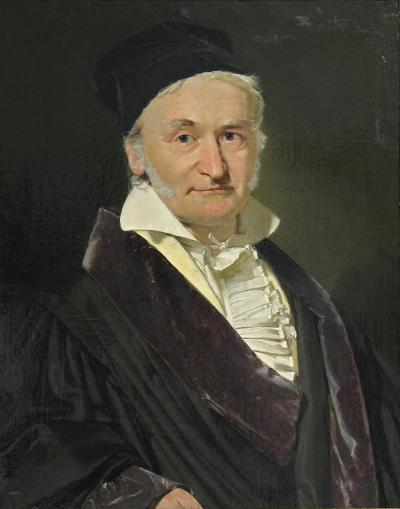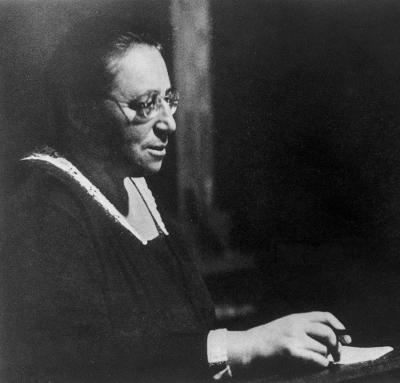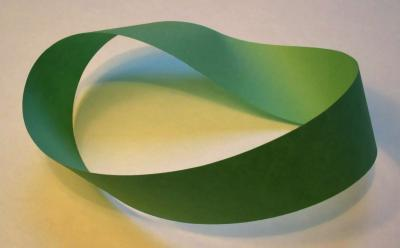Mathematician Test

This is a fun test in which examines your knowledge and enthusiasm in mathematics.
In order to achieve an honest result, do not use internet to find an answer.

1)

In which interval does your age fall into?
(This question doesn't have score)

2)

(This question doesn't have score)

3)

How much do you like mathematics?

4)
 Do you spend any of your free time on math?   Nope.   Ofcourse!
5)

What does your job have to do with mathematics?

6)

What does your future job have to do with mathematics?

7)Who is the man in the picture?

8)Who is the woman in the picture?

9)What did he achieve as a mathematician?

10)

How many answers does this equation have?
(real or otherwise)
x^3 + 1 = 0

11)

Rational numbers are a dense subset of real numbers.

12)

What is the field of Group theory about?

13)

Derivative enters a bar, threatens to injure all the functions. Exponential function comes forward to confront it. Why isn't exponential scared?

14)What is the object in the picture called?

15)What is the object in the picture called?

16)

We want to choose six students from a class of ten, then put four of them on a table in circle. How many are the possible ways?
(You are free to use calculator)

17)

Do you normally talk math with people?

18)